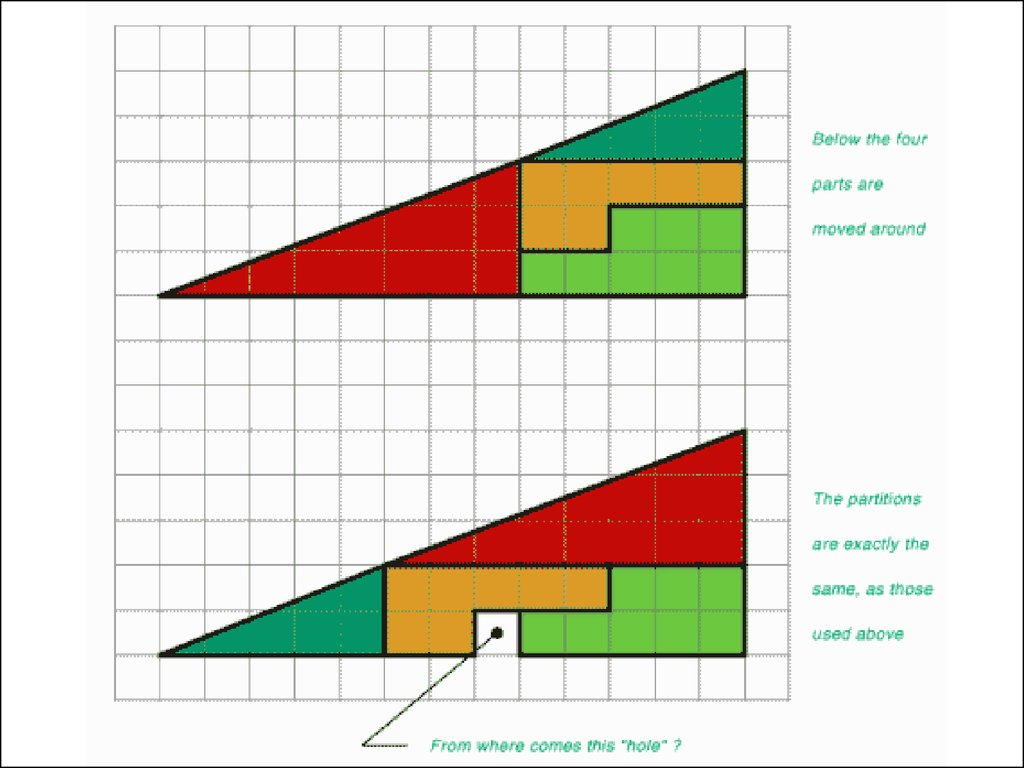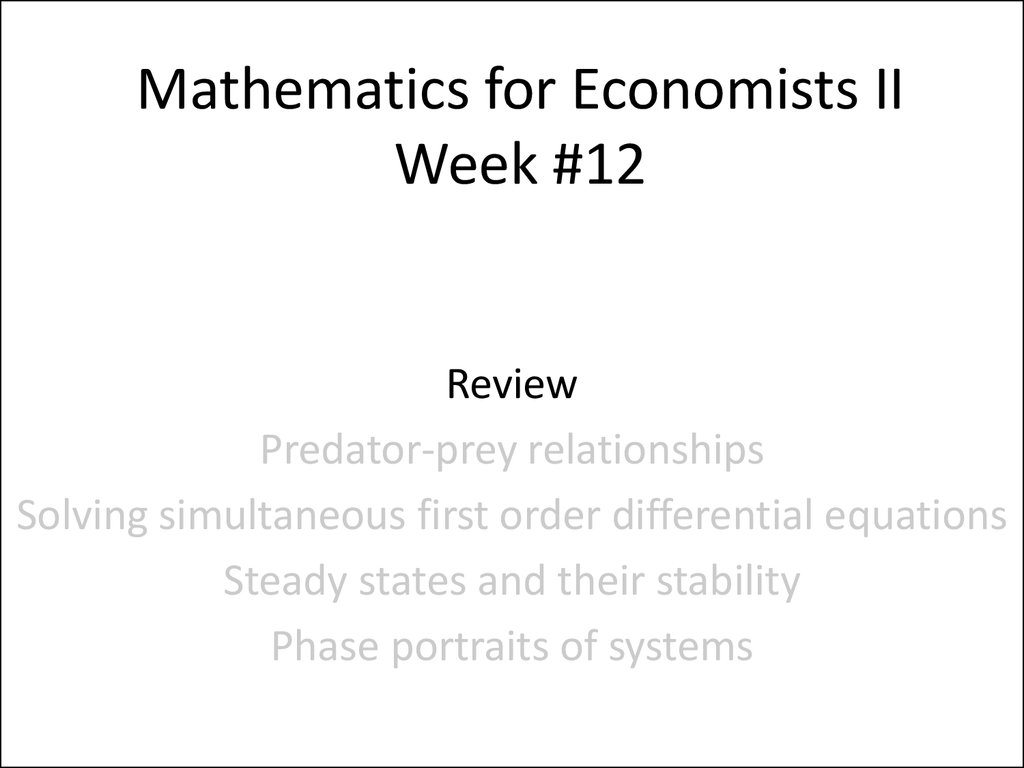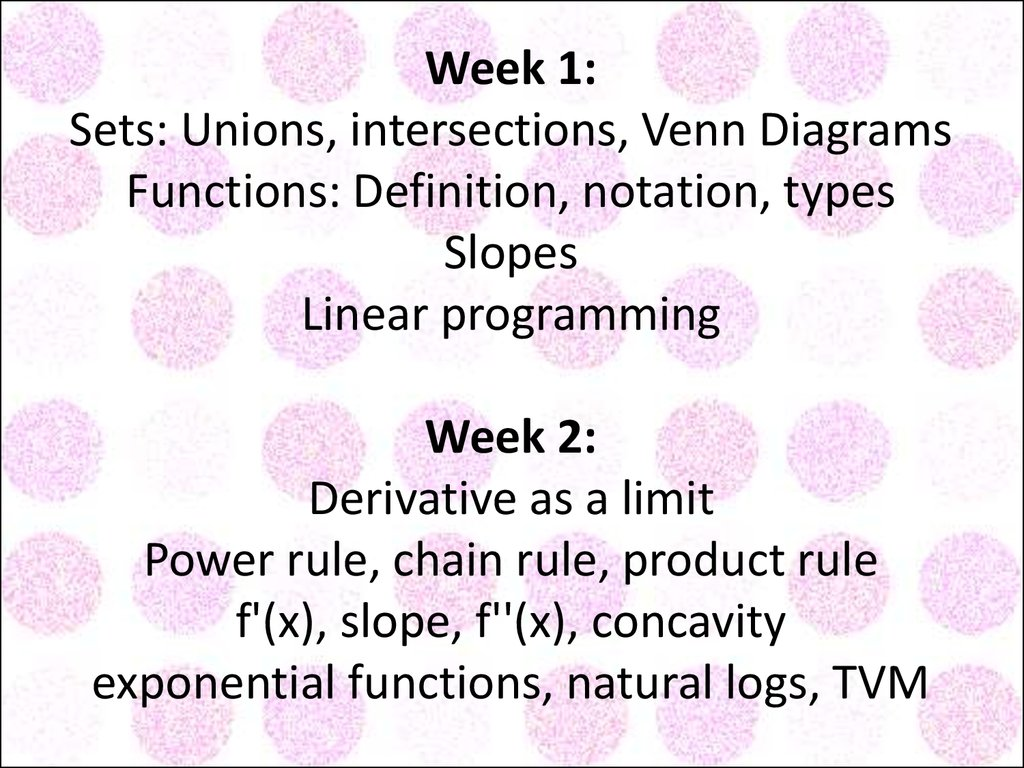# Mathematics for еconomists. (Week 1-12)

## 2. Mathematics for Economists II Week #12

Review
Predator-prey relationships
Solving simultaneous first order differential equations
Phase portraits of systems

## 4. Weeks 3 and 4: Solve simultaneous equations Writing equations in matrix form: Ax = B Rules for adding, subtracting, multiplying matrices Identity matrix: Iij=1 if i=j, and 0 if i≠j Rank (How many "leading ones"?) Row operations Transposed matrices Calcu

Weeks 3 and 4:
Solve simultaneous equations
Writing equations in matrix form: Ax = B
Rules for adding, subtracting, multiplying matrices
Identity matrix: Iij=1 if i=j, and 0 if i≠j
Row operations
Transposed matrices
Calculating determinants
Testing for invertibility and calculating A-1
Cramer's Rule
Eigenvalues

Weeks 3 and 4:
Example:
2 1
1 2
Eigenvalues

## 7. Weeks 3 and 4: Example: [■8(2&[email protected]&2)] (2 - i)(2 - i) - 1 = 0 i2 - 4i + 4 - 1 = 0 i2 - 4i + 3 = 0 (i - 3)(i - 1) = 0, so i = 1, 3 Eigenvalues

Weeks 3 and 4:
Example:
2 1
1 2
(2 - i)(2 - i) - 1 = 0
i2 - 4i + 4 - 1 = 0
i2 - 4i + 3 = 0
(i - 3)(i - 1) = 0, so i = 1, 3
Eigenvalues

## 8. Now... the vectors. Example: i = 1, 3 [■8(2&[email protected]&2)][■8([email protected])]=1[■8([email protected])], leading to x = -y [■8(2&[email protected]&2)][■8([email protected])]=3[■8([email protected])], leading to x = y Eigenvectors are v1 = [■8([email protected])], v2 = [■8([email protected])]

Now... the vectors.
Example:
i = 1, 3